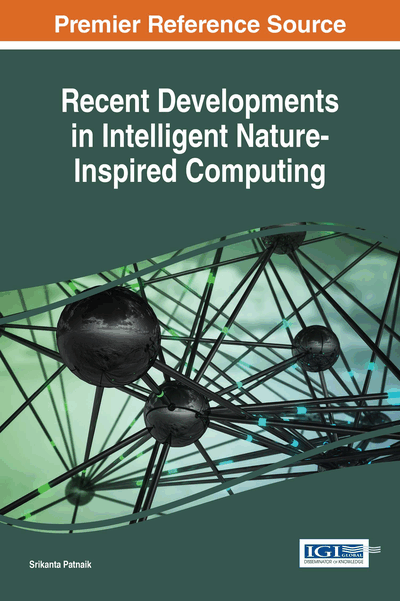# Stellar Mass Black Hole for Engineering Optimization

Premalatha Kandhasamy (Bannari Amman Institute of Technology, India), Balamurugan R (Bannari Amman Institute of Technology, India) and Kannimuthu S (Karpagam College of Engineering, India)
DOI: 10.4018/978-1-5225-2322-2.ch003

## Abstract

In recent years, nature-inspired algorithms have been popular due to the fact that many real-world optimization problems are increasingly large, complex and dynamic. By reasons of the size and complexity of the problems, it is necessary to develop an optimization method whose efficiency is measured by finding the near optimal solution within a reasonable amount of time. A black hole is an object that has enough masses in a small enough volume that its gravitational force is strong enough to prevent light or anything else from escaping. Stellar mass Black hole Optimization (SBO) is a novel optimization algorithm inspired from the property of the gravity's relentless pull of black holes which are presented in the Universe. In this paper SBO algorithm is tested on benchmark optimization test functions and compared with the Cuckoo Search, Particle Swarm Optimization and Artificial Bee Colony systems. The experiment results show that the SBO outperforms the existing methods.
Chapter Preview
Top

## Introduction

The objective of a heuristic approach is to generate a solution to the problem in a reasonable time frame and it is good enough to solve the problem within the search space. This solution may not be the best of all the actual solutions to the problem or it may approximate the exact solution. But it is valuable because it does not require a prohibitively long time for findings. An optimization is selecting the best solution with respect to certain criteria from the set of available solutions. Optimization problems consist of maximizing or minimizing a function by logically choosing input parameter values within a permissible set and computing the value of the function. Optimization means for a given defined domain finding the best available values of some objective function (Diewert, 2008). An optimization problem can be represented as Let f: XR from some set S to the real numbers R Let X be a subset of the Rn, often specified by a set of constraints, inequalities or equalities that the members of X have to satisfy. The domain X of f is called the search space, while the elements of X are called candidate solutions or feasible solutions. The function f is said to be an objective function or fitness function. A feasible solution that minimizes or maximizes the objective function is called an optimal solution (Newman, 2008). An element x* in X such that f(x*) ≤ f(x) for all x in X for minimization and x* is a global minimum point or such that f(x*) ≥ f(x) for all x in X for maximization and x* is global maximum point.

In domain X, f is said to have a local maximum point at the point x if there exists some ε > 0 such that f(x) ≥ f(x) for all x in X within distance ε of x. Likewise, the function has a local minimum point at x if f(x) ≤ f(x) for all x in X within distance ε of x. A global maximum point is always a local maximum point and global minimum point is always a local minimum points. A metaheuristic as an iterative generation process which guides a subordinate heuristic by combining intelligently different concepts and learning strategies are used in order to find efficiently near-optimal solutions (Osman & Laporte, 1996). A metaheuristic algorithm must be able to rapidly converge to the global optimum solution of the related objective function (Yang, 2006). Furthermore, the run-time required by a metaheuristic algorithm to reach to a global optimum solution must be at adequate levels for practical applications. The algorithmic structure of a metaheuristic algorithm is preferred to be simple enough to allow for its easy adaptation to different problems. The metaheuristic algorithm desired to has no algorithmic control parameters or very few algorithmic control parameters excluding the general parameters such as size of the population, total number of iterations and problem dimension.

Exploration and exploitation are two basic strategies during searching for the global optimum in metaheuristic optimization algorithms (Rashedi et al, 2009). The exploration process does well in enabling the algorithm to reach the best local solutions within the search space. The exploitation process succeeds the ability to reach the global optimum solution which is on the point around the local solutions obtained. Nature has rich source of inspiration and researchers have been motivated in many ways. At present, most of the new algorithms have been inspired by nature and they have been developed by enchanting inspiration from nature. In this work a novel stellar mass black hole optimization algorithm is developed for NP-hard problems based on the characteristics of stellar mass black hole. The sources of inspiration come from physical properties absorption, emission, coalescing and vanishing of black hole.

## Complete Chapter List

Search this Book:
Reset